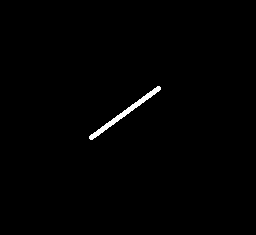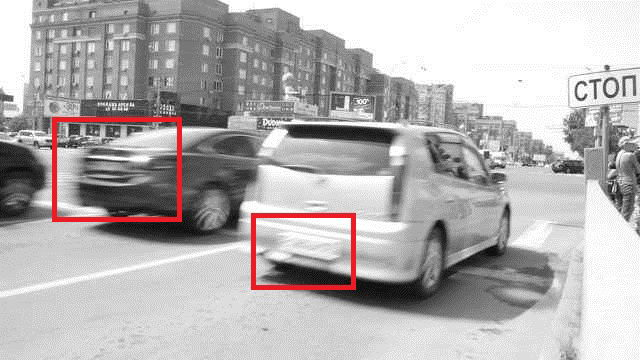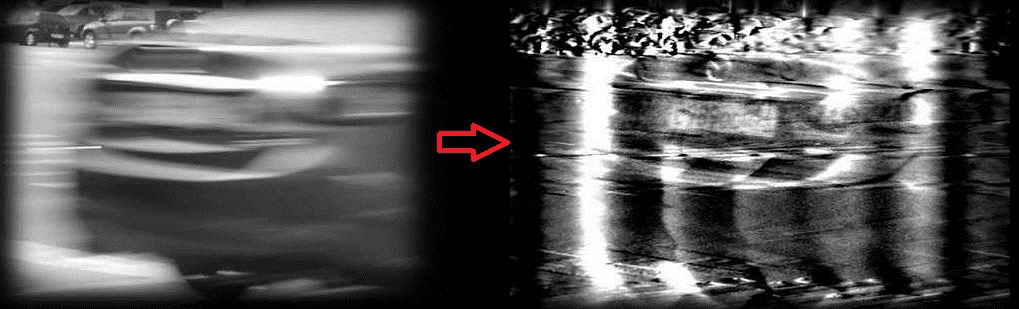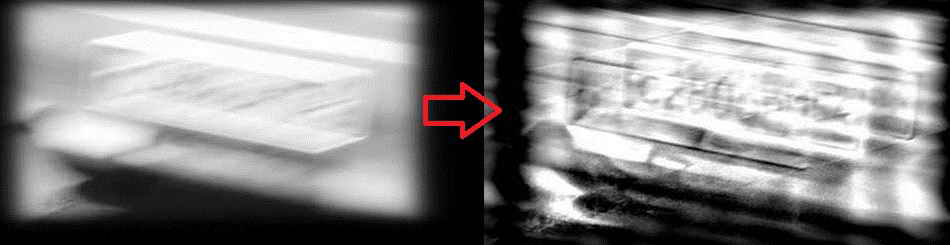OpenCV  4.0.1 Open Source Computer Vision
Motion Deblur Filter

## Goal

In this tutorial you will learn:

• what the PSF of a motion blur image is
• how to restore a motion blur image

## Theory

For the degradation image model theory and the Wiener filter theory you can refer to the tutorial Out-of-focus Deblur Filter. On this page only a linear motion blur distortion is considered. The motion blur image on this page is a real world image. The blur was caused by a moving subject.

### What is the PSF of a motion blur image?

The point spread function (PSF) of a linear motion blur distortion is a line segment. Such a PSF is specified by two parameters: $$LEN$$ is the length of the blur and $$THETA$$ is the angle of motion.Point spread function of a linear motion blur distortion

### How to restore a blurred image?

On this page the Wiener filter is used as the restoration filter, for details you can refer to the tutorial Out-of-focus Deblur Filter. In order to synthesize the Wiener filter for a motion blur case, it needs to specify the signal-to-noise ratio ( $$SNR$$), $$LEN$$ and $$THETA$$ of the PSF.

## Source code

You can find source code in the samples/cpp/tutorial_code/ImgProc/motion_deblur_filter/motion_deblur_filter.cpp of the OpenCV source code library.

#include <iostream>
using namespace cv;
using namespace std;
void help();
void calcPSF(Mat& outputImg, Size filterSize, int len, double theta);
void fftshift(const Mat& inputImg, Mat& outputImg);
void filter2DFreq(const Mat& inputImg, Mat& outputImg, const Mat& H);
void calcWnrFilter(const Mat& input_h_PSF, Mat& output_G, double nsr);
void edgetaper(const Mat& inputImg, Mat& outputImg, double gamma = 5.0, double beta = 0.2);
const String keys =
"{help h usage ? | | print this message }"
"{image |input.png | input image name }"
"{LEN |125 | length of a motion }"
"{THETA |0 | angle of a motion in degrees }"
"{SNR |700 | signal to noise ratio }"
;
int main(int argc, char *argv[])
{
help();
CommandLineParser parser(argc, argv, keys);
if (parser.has("help"))
{
parser.printMessage();
return 0;
}
int LEN = parser.get<int>("LEN");
double THETA = parser.get<double>("THETA");
int snr = parser.get<int>("SNR");
string strInFileName = parser.get<String>("image");
if (!parser.check())
{
parser.printErrors();
return 0;
}
Mat imgIn;
if (imgIn.empty()) //check whether the image is loaded or not
{
cout << "ERROR : Image cannot be loaded..!!" << endl;
return -1;
}
Mat imgOut;
// it needs to process even image only
Rect roi = Rect(0, 0, imgIn.cols & -2, imgIn.rows & -2);
//Hw calculation (start)
Mat Hw, h;
calcPSF(h, roi.size(), LEN, THETA);
calcWnrFilter(h, Hw, 1.0 / double(snr));
//Hw calculation (stop)
imgIn.convertTo(imgIn, CV_32F);
edgetaper(imgIn, imgIn);
// filtering (start)
filter2DFreq(imgIn(roi), imgOut, Hw);
// filtering (stop)
imgOut.convertTo(imgOut, CV_8U);
normalize(imgOut, imgOut, 0, 255, NORM_MINMAX);
imwrite("result.jpg", imgOut);
return 0;
}
void help()
{
cout << "2018-08-14" << endl;
cout << "Motion_deblur_v2" << endl;
cout << "You will learn how to recover an image with motion blur distortion using a Wiener filter" << endl;
}
void calcPSF(Mat& outputImg, Size filterSize, int len, double theta)
{
Mat h(filterSize, CV_32F, Scalar(0));
Point point(filterSize.width / 2, filterSize.height / 2);
ellipse(h, point, Size(0, cvRound(float(len) / 2.0)), 90.0 - theta, 0, 360, Scalar(255), FILLED);
Scalar summa = sum(h);
outputImg = h / summa;
}
void fftshift(const Mat& inputImg, Mat& outputImg)
{
outputImg = inputImg.clone();
int cx = outputImg.cols / 2;
int cy = outputImg.rows / 2;
Mat q0(outputImg, Rect(0, 0, cx, cy));
Mat q1(outputImg, Rect(cx, 0, cx, cy));
Mat q2(outputImg, Rect(0, cy, cx, cy));
Mat q3(outputImg, Rect(cx, cy, cx, cy));
Mat tmp;
q0.copyTo(tmp);
q3.copyTo(q0);
tmp.copyTo(q3);
q1.copyTo(tmp);
q2.copyTo(q1);
tmp.copyTo(q2);
}
void filter2DFreq(const Mat& inputImg, Mat& outputImg, const Mat& H)
{
Mat planes = { Mat_<float>(inputImg.clone()), Mat::zeros(inputImg.size(), CV_32F) };
Mat complexI;
merge(planes, 2, complexI);
dft(complexI, complexI, DFT_SCALE);
Mat planesH = { Mat_<float>(H.clone()), Mat::zeros(H.size(), CV_32F) };
Mat complexH;
merge(planesH, 2, complexH);
Mat complexIH;
mulSpectrums(complexI, complexH, complexIH, 0);
idft(complexIH, complexIH);
split(complexIH, planes);
outputImg = planes;
}
void calcWnrFilter(const Mat& input_h_PSF, Mat& output_G, double nsr)
{
Mat h_PSF_shifted;
fftshift(input_h_PSF, h_PSF_shifted);
Mat planes = { Mat_<float>(h_PSF_shifted.clone()), Mat::zeros(h_PSF_shifted.size(), CV_32F) };
Mat complexI;
merge(planes, 2, complexI);
dft(complexI, complexI);
split(complexI, planes);
Mat denom;
pow(abs(planes), 2, denom);
denom += nsr;
divide(planes, denom, output_G);
}
void edgetaper(const Mat& inputImg, Mat& outputImg, double gamma, double beta)
{
int Nx = inputImg.cols;
int Ny = inputImg.rows;
Mat w1(1, Nx, CV_32F, Scalar(0));
Mat w2(Ny, 1, CV_32F, Scalar(0));
float* p1 = w1.ptr<float>(0);
float* p2 = w2.ptr<float>(0);
float dx = float(2.0 * CV_PI / Nx);
float x = float(-CV_PI);
for (int i = 0; i < Nx; i++)
{
p1[i] = float(0.5 * (tanh((x + gamma / 2) / beta) - tanh((x - gamma / 2) / beta)));
x += dx;
}
float dy = float(2.0 * CV_PI / Ny);
float y = float(-CV_PI);
for (int i = 0; i < Ny; i++)
{
p2[i] = float(0.5 * (tanh((y + gamma / 2) / beta) - tanh((y - gamma / 2) / beta)));
y += dy;
}
Mat w = w2 * w1;
multiply(inputImg, w, outputImg);
}

## Explanation

A motion blur image recovering algorithm consists of PSF generation, Wiener filter generation and filtering a blurred image in a frequency domain:

// it needs to process even image only
Rect roi = Rect(0, 0, imgIn.cols & -2, imgIn.rows & -2);
//Hw calculation (start)
Mat Hw, h;
calcPSF(h, roi.size(), LEN, THETA);
calcWnrFilter(h, Hw, 1.0 / double(snr));
//Hw calculation (stop)
imgIn.convertTo(imgIn, CV_32F);
edgetaper(imgIn, imgIn);
// filtering (start)
filter2DFreq(imgIn(roi), imgOut, Hw);
// filtering (stop)

A function calcPSF() forms a PSF according to input parameters $$LEN$$ and $$THETA$$ (in degrees):

void calcPSF(Mat& outputImg, Size filterSize, int len, double theta)
{
Mat h(filterSize, CV_32F, Scalar(0));
Point point(filterSize.width / 2, filterSize.height / 2);
ellipse(h, point, Size(0, cvRound(float(len) / 2.0)), 90.0 - theta, 0, 360, Scalar(255), FILLED);
Scalar summa = sum(h);
outputImg = h / summa;
}

A function edgetaper() tapers the input image’s edges in order to reduce the ringing effect in a restored image:

void edgetaper(const Mat& inputImg, Mat& outputImg, double gamma, double beta)
{
int Nx = inputImg.cols;
int Ny = inputImg.rows;
Mat w1(1, Nx, CV_32F, Scalar(0));
Mat w2(Ny, 1, CV_32F, Scalar(0));
float* p1 = w1.ptr<float>(0);
float* p2 = w2.ptr<float>(0);
float dx = float(2.0 * CV_PI / Nx);
float x = float(-CV_PI);
for (int i = 0; i < Nx; i++)
{
p1[i] = float(0.5 * (tanh((x + gamma / 2) / beta) - tanh((x - gamma / 2) / beta)));
x += dx;
}
float dy = float(2.0 * CV_PI / Ny);
float y = float(-CV_PI);
for (int i = 0; i < Ny; i++)
{
p2[i] = float(0.5 * (tanh((y + gamma / 2) / beta) - tanh((y - gamma / 2) / beta)));
y += dy;
}
Mat w = w2 * w1;
multiply(inputImg, w, outputImg);
}

The functions calcWnrFilter(), fftshift() and filter2DFreq() realize an image filtration by a specified PSF in the frequency domain. The functions are copied from the tutorial Out-of-focus Deblur Filter.

## Result

Below you can see the real world image with motion blur distortion. The license plate is not readable on both cars. The red markers show the car’s license plate location.Motion blur image. The license plates are not readable

Below you can see the restoration result for the black car license plate. The result has been computed with $$LEN$$ = 125, $$THETA$$ = 0, $$SNR$$ = 700.The restored image of the black car license plate

Below you can see the restoration result for the white car license plate. The result has been computed with $$LEN$$ = 78, $$THETA$$ = 15, $$SNR$$ = 300.The restored image of the white car license plate

The values of $$SNR$$, $$LEN$$ and $$THETA$$ were selected manually to give the best possible visual result. The $$THETA$$ parameter coincides with the car’s moving direction, and the $$LEN$$ parameter depends on the car’s moving speed. The result is not perfect, but at least it gives us a hint of the image’s content. With some effort, the car license plate is now readable.

Note
The parameters $$LEN$$ and $$THETA$$ are the most important. You should adjust $$LEN$$ and $$THETA$$ first, then $$SNR$$.

You can also find a quick video demonstration of a license plate recovering method YouTube.